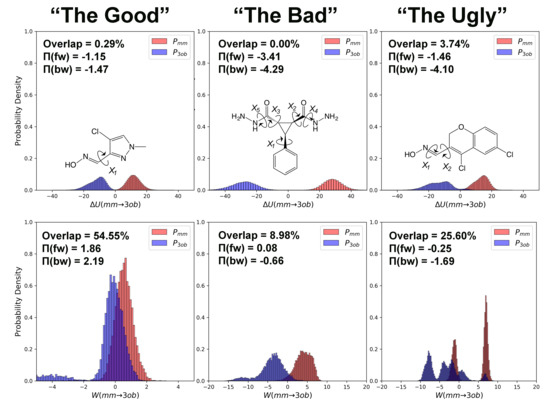Previous Article in Journal
Bioactivity Profile of the Diterpene Isosteviol and its Derivatives
Previous Article in Special Issue
Quantum Mechanical/Molecular Mechanical Analysis of the Catalytic Mechanism of Phosphoserine Phosphatase
Open AccessFeature PaperArticle

# The Good, the Bad, and the Ugly: “HiPen”, a New Dataset for Validating (S)QM/MM Free Energy Simulations

byFiona L. Kearns 1,Luke Warrensford 1,Stefan Boresch 2,*andH. Lee Woodcock 1,*1
Department of Chemistry, University of South Florida, 4202 E. Fowler Avenue, Tampa, FL 33620, USA
2
Department of Computational Biological Chemistry, Faculty of Chemistry, University of Vienna, Waehringerstrasse 17, A-1090 Vienna, Austria
*
Authors to whom correspondence should be addressed.
Molecules 2019, 24(4), 681; https://doi.org/10.3390/molecules24040681
Received: 27 December 2018 / Revised: 28 January 2019 / Accepted: 29 January 2019 / Published: 14 February 2019
Indirect (S)QM/MM free energy simulations (FES) are vital to efficiently incorporating sufficient sampling and accurate (QM) energetic evaluations when estimating free energies of practical/experimental interest. Connecting between levels of theory, i.e., calculating $Δ A l o w → h i g h$ , remains to be the most challenging step within an indirect FES protocol. To improve calculations of $Δ A l o w → h i g h$ , we must: (1) compare the performance of all FES methods currently available; and (2) compile and maintain datasets of $Δ A l o w → h i g h$ calculated for a wide-variety of molecules so that future practitioners may replicate or improve upon the current state-of-the-art. Towards these two aims, we introduce a new dataset, “HiPen”, which tabulates $Δ A g a s M M → 3 o b$ (the free energy associated with switching from an $M M$ to an $S C C − D F T B$ molecular description using the 3ob parameter set in gas phase), calculated for 22 drug-like small molecules. We compare the calculation of this value using free energy perturbation, Bennett’s acceptance ratio, Jarzynski’s equation, and Crooks’ equation. We also predict the reliability of each calculated $Δ A g a s M M → 3 o b$ by evaluating several convergence criteria including sample size hysteresis, overlap statistics, and bias metric ( $Π$ ). Within the total dataset, three distinct categories of molecules emerge: the “good” molecules, for which we can obtain converged $Δ A g a s M M → 3 o b$ using Jarzynski’s equation; “bad” molecules which require Crooks’ equation to obtain a converged $Δ A g a s M M → 3 o b$ ; and “ugly” molecules for which we cannot obtain reliably converged $Δ A g a s M M → 3 o b$ with either Jarzynski’s or Crooks’ equations. We discuss, in depth, results from several example molecules in each of these categories and describe how dihedral discrepancies between levels of theory cause convergence failures even for these gas phase free energy simulations. View Full-Text
Show FiguresGraphical abstract

MDPI and ACS Style

Kearns, F.L.; Warrensford, L.; Boresch, S.; Woodcock, H.L. The Good, the Bad, and the Ugly: “HiPen”, a New Dataset for Validating (S)QM/MM Free Energy Simulations. Molecules 2019, 24, 681.